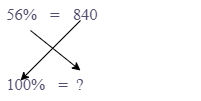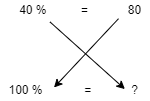# Capgemini aptitude questions

Capgemini is one of the leading IT services companies in the world. As such, they have high standards for their recruitment tests and are known for asking challenging aptitude questions. To give yourself the best chance of success, it’s important to know what to expect from Capgemini recruitment tests. Aptitude questions form a crucial part of the Capgemini recruitment tests and may include numerical tests, logical reasoning, verbal reasoning, data interpretation, and critical thinking. To help you prepare, here are some of the most common aptitude questions you may encounter during a Capgemini Interview Process:

1. Find the third proportional to 8 and 12 .

• The third proportional of a proportion is the second term of the mean terms.
• For example, if we have a:b = c:d, then the term 'c' is the third proportional to 'a' and 'b'.
• The required formula is

• Let the third proportion be x , 8:12 = 12:x

$\Rightarrow \frac{8}{12} = \frac{12}{x}$

$\Rightarrow$x = 12 $\times$ $\frac{12}{8}$

$\Rightarrow$ $x = 18.$

1. By selling 144 hens Mahesh suffer a loss equal to the selling price of 6 hens. Find his loss percent?

• loss = C.P – S.P
• S.P of 6 hen = C.P of 144 hens – S.P of 144 hens
• S.P of 150 hens = C.P of 144 hens
• $\frac{6}{150} \times 100$= 4% loss
1. 12, 18, 24, 30, 36, 42, 48, 54, 60.

What is the average of these numbers?

• Easy method : Trick 1

• Average = sum $\div$ number

• Average = $\frac {12+18+24+30+36+42+48+54+60} { 9}$

= $\frac {324} {9}$ = 36

Trick 2

• Average of continuous natural numbers, odd numbers, even numbers and multiples

$\frac{FIRST NUMBER + LAST NUMBER}{2}$

$\frac{12 + 60}{2}$ = $\frac{72}{2}$ = 36

1. In a college election in which two people competed, the man with 78% of the vote won by 840 votes. Then what is the total number of votes polled?

• Votes received by the first person = = 78% <br> Votes received by the second person = 100 -78 = 22% <br>

Percentage difference between them $\Rightarrow$ majority = 78% - 22% = 56% <br>

56 % $\rightarrow$ 840<br> 100% $\rightarrow$ ?

• To find 100%, you just need to cross-multiply them and then divide them as shown below.• Total Polled Votes = $\frac{840\times100}{56} = 1500$

1. 40% marks are required to pass an examination. A student got 60 marks but lost by 20 marks. So what is the maximum marks of the examination?

• 40% $\Rightarrow$80%<br> 100%$\Rightarrow$ ?
•∴ Total marks $= \frac{100\times80}{40}=200$